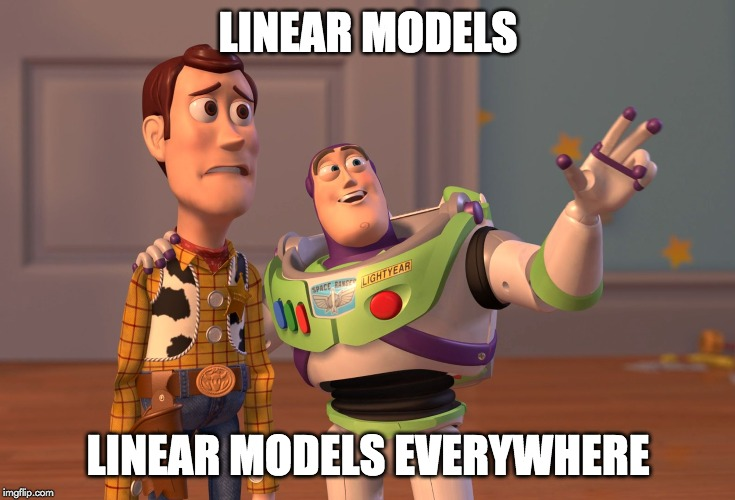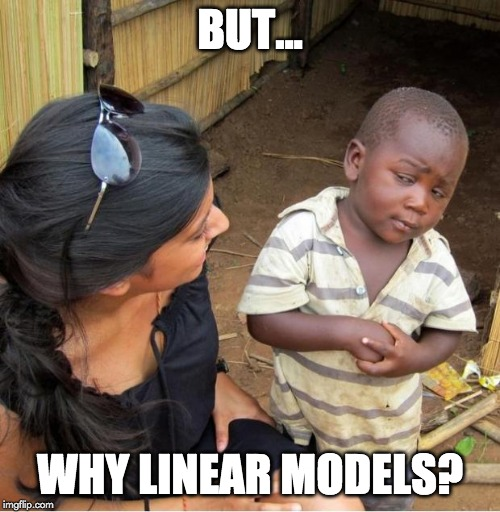# Linear Models

A 3 minute read written by
Audun Eltvik and Michael Nordmo
03.12.2019

There are many different types of machine learning models. Linear models are some of the simplest, but also some of the most widely used. In this post we’ll explain what a linear model is and why linear models are so popular.Let's say you are going on a 100 km road trip, and you want to know how much money you should expect to spend on gas. Unfortunately for you, there is no information on the fuel efficiency of your car, so you can't simply calculate the number.

Fortunately for you, however, you have recorded the distance traveled and gas usage for previous roadtrips. Using this data, it might be possible to build a model that can be used to predict roughly how much gas you would expect to need for your road trip.

The first step in any machine learning problem is usually to visualize the data in some way. In this case, we plot distance traveled vs gas usage in a scatter plot.In machine learning, we decide on some model that we believe describes the data. This model is then fitted to the data, and used to extrapolate to new situations. The simplest of such models is the humble line. A line, or more accurately a linear model, assumes that the relationship between the input and the output is such that an increase in input produces the same increase in output multiplied by some constant. More precisely, is is a parameterized model of the form

Y = a*X + b

Where X is the input (distance traveled), Y is the output (gas usage) and a and b are parameters (the relationship between distance traveled and gas usage) to be determined using machine learning. The perceptive reader will notice that it’s not possible to run a line through all the points in the above image. So we need some sense of a best line.

The idea of a best model in machine learning is captured by the cost function. This is a function of the parameters which measures how “bad” your model is performing on the given data, with zero being a perfect model. The model is trained on your data set by minimizing the cost function, which corresponds to finding the model parameters that performs best on your data.

For this situation, a good cost function is the sum of the distances between the line and the points. The square of the distance is often used to measure the error for each individual data point and, hence, the method is known as the least squares method. The image below shows the line that represents the linear model you get when applying the least squares method to the problem above. Using this model, we can predict what the gas usage will be for a given distance. For 100 km, for instance, the model predicts that we will use about 6.7 liters of gasAs an informal definition, we can say that linear model are all models that represents the relationships in the data using only straight lines.There are many reasons why linear models are widely used, but we will mention three important ones here. Firstly, linear models are relatively easy to interpret. The model parameters may be used to gain insights into the data and to explain why a machine learning model made a particular prediction. Interpretability is a very attractive quality in many data science problems.

Secondly, linear models have measures for uncertainty. The linear regression model in the example above have well defined ways of calculating confidence intervals and prediction intervals. Confidence intervals tells you how uncertain the model is about the parameters it has learned from the data. Prediction intervals tells you how uncertain the model is about a particular prediction. Machine learning models are prone to errors and having a measure of uncertainty is often very valuable for a data scientist.

A third reason is that linear models may be extended to fit many complex nonlinear relationships. The data scientist has to decide which nonlinear relationships to model, but if the relationships in the data are not too complex, a linear model will often suffice. If the relationships in your data are very complex and nonlinear, however, other machine learning models may be better suited.